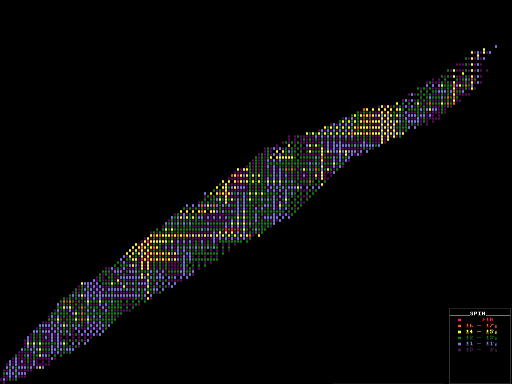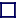Segre Chart Colour-Coded by SPIN [Pop Out Page]

### GROUND-STATE NUCLEAR SPIN

Nuclei with filled nuclear shells (with a magic number of both protons and of neutrons) are spherical. Those with un-filled nuclear shells are non-spherical, either oblate or prolate spheroids.

Nucleons (protons and neutrons) have half integral spin (of plus or minus a half). Within a nucleus, the nucleons have a strong tendency to pair up as best they can subject to shell constraints, neutron with neutron and proton with proton, so that their spins cancel. Thus for all even-Z even-N nuclei (like carbon-12) the ground state spin is always zero. The nuclear spin is the total angular momentum, which is the vector sum of the spin angular momentum plus the orbital angular momentum (or rotational spin of the whole nucleus). Some nucleons pair up spins anti-parallel to become spin-0 units, whilst others can remain unpaired. Thus the ground state spin of some nuclides can be quite large, up to 11/2. The ground state spin is the lowest energy spin state, and is only zero for even-Z even-N nuclei.

The spin is either integral (bosonic) or half-integral (fermionic), depending upon whether the number of nucleons is an odd or even number; the even numbers giving rise to integral spins. The ground state spin for all even-N even-Z nuclei is always zero, but for other nuclei the total spin is not necessarily the lowest value after pairing-off, as some spins remain un-paired giving total spins as high as 10. The spin-½ nucleons in even-Z even-N nuclei pair together with the spins aligned anti-parallel into spin-zero bosons, producing a nucleus with an overall spin of zero. Being in the ground state, these then undergo Bose-Einstein Condensation into Cooper-pairs resulting in a nucleus that is superfluid. This is why the nuclei of even-Z even-N nuclides are particularly stable.